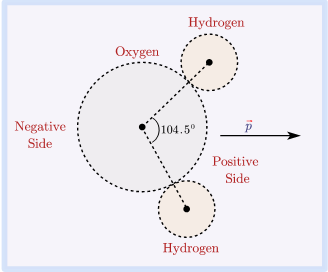# Electric Dipole and Dipole Moment

## What is an Electric Dipole

• Electric dipole is a pair of equal and opposite charges, $+q$ and $-q$, separated by a very small distance..
• Total charge of the dipole is zero but electric field of the dipole is not zero as charges q and -q are separated by some distance and electric field due to them when added is not zero.
• Examples of electric dipole:- Dipoles are common in nature. Molecules like
$H_2O,\,\, HCl,\,\, CH_3COOH$
are electric dipoles and have permanent dipole moments. They have permanent dipole moments because the center of their positive charges does not fall exactly over the center of their negative charges.Figure given below shows molecule of water.Center of mass of negative and positive charges does not coincide in a water molecule In this figure the electric dipole moment $\vec{p}$ points from negative or oxygen side to the positive or hydrogen side of the molecule.

### Physical significance of Electric Dipole and dipole moment

• Atoms as a whole are electrically neutral in their ground state. We know that atoms have equal amount of positive and negative charge. Similar to atoms molecules are also neutral but they also have equal amount of positive and negative charges.
• Now when in a system, algebraic sum of all the charges is zero it does not necessarily mean that electric field produced by the system is zero everywhere. This makes study of electric dipoles important for electrical phenomenon in matter.
• Matter which is made up of atoms and molecules is electrically neutral. If the center of mass of positive charges coincides with that of negative charges then molecule behaves as non-polar molecule. On the other hand, if center of mass of positive charges does not coincides with that of negative charges then molecule behaves as polar molecule. These polar molecules have permanent dipole moments. These dipole moments are randomly oriented in the absence of external electric field. If we place a material with polar molecules in external electric field then these molecules align themselves in the direction of the field. This results in the development of a net dipole moment. This particular piece of material is said to be polarized.
• So study of dipole and dipole moments gives a measure of the polarization of a net neutral system. The study of dipole moments measures the tendency of a dipole to align with an external electric field.

Electric dipole moment occurs when there is a separation of charge. It can occur in electrically neutral molecules with ionic bonds or molecules with covalent bonds. Dipole moments measures the electric polarity of system of charges.

Electric dipole moment measures the strength of an electric dipole. It is a vector quantity.

Electric dipole moment definition :- The dipole moment of an electric field is a vector whose magnitude is charge times the separation between two opposite charges.

• Direction of dipole moment is along the dipole axis from negative charge to positive charge.
• Consider the figure given below which shows an electric dipole consisting of charges $(\pm q)$ separated by a small distance $2a$.
Mathematically, \begin{align*} \text{Dipole Moment}=&\text{either one of charges}\times \\ &\text{separation vector from +ive to -ive} \end{align*} $\vec{p}=q(2\vec{a})C.m$ Above equation gives Electric dipole moment formula
• Magnitude of dipole moment is
$|\vec{p}|=q\times 2a$
• The Electric dipole moment direction is from negative charge to positive charge.
• The SI unit of dipole moment is Coulomb-meter$(C-m)$

### What is an ideal electric dipole?

Answer: If charge $q$ gets larger and the distance $2a$ gets smaller and smaller such that the dipole moment, $p=2\times 2a$ has finite value then such a dipole of negligibly small size is called an ideal or point dipole

### Frequently asked questions on electric dipole and electric dipole moment

Question 1: What is the difference between electric dipole and electric dipole moment?
Answer: Electric dipole is just the separation of two equal and opposite charges separated a small distance whereas electric dipole moment measures the strength of an electric dipole. Electric dipole moment occurs due to the existence of an electric dipole. It measure the electric polarity of the system of charges.
Question 2: How is electric dipole calculated?
Answer: We do not calculate electric dipole. Electric dipole is just a formation of two equal and opposite charges. We rather calculate its dipole moment.
Question 3: What is the significance of electric dipole?
Question 4: Is electric dipole moment scalar or vector?
Answer: It is a vector quantity.
Question 5: Why electric dipole moment is from negative to positive?
Answer: This is purely a matter of convention (read more here). If you want you can define it other way around but it might lead to change in equation for energy, torque etc..